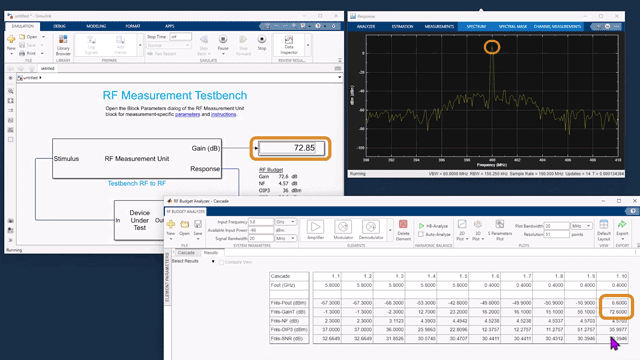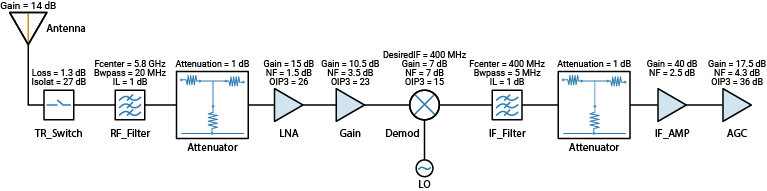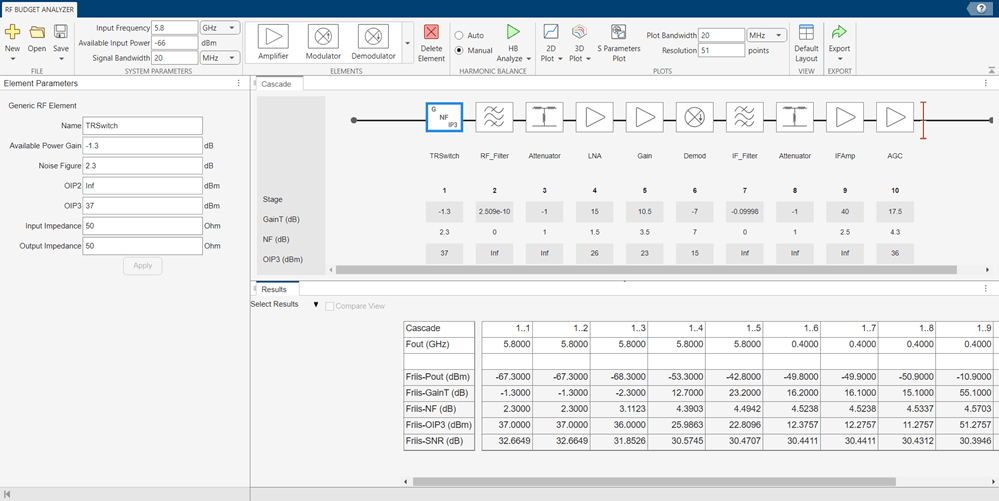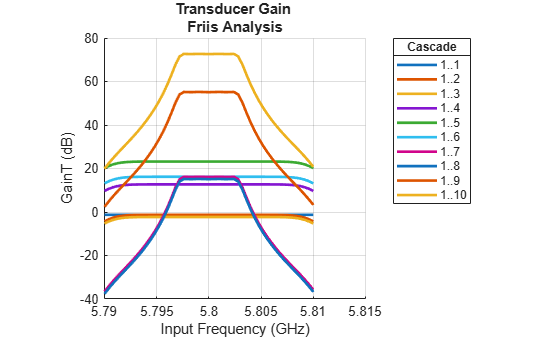# Superheterodyne Receiver Using RF Budget Analyzer App

This example shows how to build a superheterodyne receiver and analyze the receiver's RF budget for gain, noise figure, and IP3 using the RF Budget Analyzer app. The receiver is a part of a transmitter-receiver system described in the IEEE conference papers,  and .

### Introduction

RF system designers begin the design process with a budget specification for how much gain, noise figure (NF), and nonlinearity (IP3) the entire system must satisfy. To assure the feasibility of an architecture modeled as a simple cascade of RF elements, designers calculate both the per-stage and cascade values of gain, noise figure and IP3 (third-intercept point).

Using the RF Budget Analyzer app, you can:

• Build a cascade of RF elements.

• Calculate the per-stage and cascade output power, gain, noise figure, SNR, and IP3 of the system.

• Export the per-stage and cascade values to the MATLAB™ workspace.

• Export the system design to RF Blockset for simulation.

• Export the system design to RF Blockset measurement testbench as a DUT (device under test) subsystem and verify the results obtained using the app.

### System Architecture

The receiver system architecture designed using the app is:The receiver bandwidth is between 5.825 GHz and 5.845 GHz.

You can build all the components of the superheterodyne receiver using MATLAB command line and view the analysis using the RF Budget Analyzer app.

The first components in the superheterodyne receiver system architecture are the antenna and TR switch. We replace the antenna block with the effective power reaching the switch.

1. The system uses the TR switch to switch between the transmitter and the receiver. The switch adds a loss of 1.3 dB to the system. Create a `TRSwitch` with a gain of -1.3 dB, and OIP3 of 37 dBm. To match the RF budget results from reference , the noise figure is assumed to be 2.3 dB.

```elements(1) = rfelement('Name','TRSwitch','Gain',-1.3,'NF',2.3,'OIP3',37); ```

2. To model the RF bandpass filter use `rffilter` to design the filter. See also example - Design IF Butterworth Bandpass Filter. From the above example, Zout of the filter is found to be 132.986 Ohms. But for budget calculation, each stage is terminated by 50 Ohms internally. Therefore, to achieve an insertion loss of 1 dB, the Zin of the next element, i.e., amplifier, is set to 132.896 Ohms.

```Fcenter = 5.8e9; Bwpass = 20e6; Z = 132.986; elements(2) = rffilter('ResponseType','Bandpass', ... 'FilterType','Butterworth','FilterOrder',6, ... 'PassbandAttenuation',10*log10(2), ... 'Implementation','Transfer function', ... 'PassbandFrequency',[Fcenter-Bwpass/2 Fcenter+Bwpass/2],'Zout',50, ... 'Name','RF_Filter'); ```

The S-parameters for this filter are not ideal and automatically inserts a loss of approximately -1dB into the system.

3. Use the `amplifier` object to model a `Low Noise Amplifier` block with a gain of 15 dB, noise figure of 1.5 dB, and OIP3 of 26 dBm.

```elements(3) = amplifier( 'Name','LNA','Gain',15,'NF',1.5,'OIP3',26, ... 'Zin',Z); ```

4. Model a `Gain` block with a gain of 10.5 dB, noise figure of 3.5 dB, and OIP3 of 23 dBm.

```elements(4) = amplifier( 'Name','Gain','Gain',10.5,'NF',3.5,'OIP3',23); ```

5. The receiver downconverts the RF frequency to an IF frequency of 400 MHz. Use the `modulator` object to create Demodulator block with a LO (Local Oscillator) frequency of 5.4 GHz, gain of -7 dB, noise figure of 7 dB, and OIP3 of 15 dBm.

```elements(5) = modulator('Name','Demod','Gain',-7,'NF',7,'OIP3',15, ... 'LO',5.4e9, 'ConverterType','Down'); ```

6. To model the RF bandpass filter use `rffilter` to design the filter.

```Fcenter = 400e6; Bwpass = 5e6; elements(6) = rffilter('ResponseType','Bandpass', ... 'FilterType','Butterworth','FilterOrder',4, ... 'PassbandAttenuation',10*log10(2), ... 'Implementation','Transfer function', ... 'PassbandFrequency',[Fcenter-Bwpass/2 Fcenter+Bwpass/2],'Zout',50, ... 'Name','IF_Filter'); ```

The S-parameters for this filter are not ideal and automatically inserts a loss of approximately -1dB into the system.

7. Model an `IF Amplifier` block with a gain of 40 dB and a noise figure of 2.5 dB.

```elements(7) = amplifier( 'Name','IFAmp','Gain',40,'NF',2.5,'Zin',Z); ```

8. As seen in the references, the receiver uses an AGC (Automatic Gain Control) block where the gain varies with the available input power level. For an input power of -80 dB, the AGC gain is at a maximum of 17.5 dB. Use an Amplifier block to model an AGC. Model an `AGC` block with a gain of 17.5 dB, noise figure of 4.3 dB, and OIP3 of 36 dBm.

```elements(8) = amplifier('Name','AGC','Gain',17.5,'NF',4.3,'OIP3',36); ```

9. Calculate the rbudget of the superheterodyne receiver using the following `System Parameters`: 5.8 GHz for `Input frequency`, -80 dB for `Available input power`, and 20 MHz for `Signal bandwidth`. Replace the antenna element with the effective `Available input power` which is estimated to be -66 dB reaching the TRswitch

```superhet = rfbudget( 'Elements',elements,'InputFrequency',5.8e9, ... 'AvailableInputPower',-66,'SignalBandwidth',20e6) ```
```superhet = rfbudget with properties: Elements: [1x8 rf.internal.rfbudget.Element] InputFrequency: 5.8 GHz AvailableInputPower: -66 dBm SignalBandwidth: 20 MHz Solver: Friis AutoUpdate: true Analysis Results OutputFrequency: (GHz) [ 5.8 5.8 5.8 5.8 0.4 0.4 0.4 0.4] OutputPower: (dBm) [-67.3 -67.3 -53.3 -42.8 -49.8 -49.8 -10.8 6.7] TransducerGain: (dB) [ -1.3 -1.3 12.7 23.2 16.2 16.2 55.2 72.7] NF: (dB) [ 2.3 2.3 3.531 3.657 3.693 3.693 3.728 3.728] IIP2: (dBm) [] OIP2: (dBm) [] IIP3: (dBm) [ 38.3 38.3 13.29 -0.3904 -3.824 -3.824 -3.824 -36.7] OIP3: (dBm) [ 37 37 25.99 22.81 12.38 12.38 51.38 36] SNR: (dB) [32.66 32.66 31.43 31.31 31.27 31.27 31.24 31.24] ```

View the analysis in the RF Budget Analyser app.

```show(superhet); ```10. The app displays the cascade values such as: output frequency of the receiver, output power, gain, noise figure, OIP3, and SNR (Signal-to- Noise-Ratio).

11. The RF Budget Analyzer app saves the model in a MAT-file format.

1. Plot the cascade transducer gain of the receiver using the function, `rfplot`

```rfplot(superhet,'GainT') view(90,0) ``````rfplot(superhet,'NF') view(90,0) ```You can also use the `Plot` button on the `RFBudgetAnalyzer` app to plot the different output values.

### Export to MATLAB Script

1. You can also export the model to MATLAB script format using the Export button or:

```h = exportScript(superhet); ```

The script opens automatically in a MATLAB Editor window.

```h.closeNoPrompt ```

### Verify Output Power and Transducer Gain Using RF Blockset Simulation

1. Use the Export button to export the receiver to RF Blockset or:

exportRFBlockset(superhet)

2. Run the RF Blockset model to calculate the Output power (dBm) and Transducer gain (dB) of the receiver. Note that the results match the Pout (dBm) and the GainT (dB) values of the receiver obtained using the RF Budget Analyzer app.3. Look under the mask of the Demodulator block. This block consists of an ideal filter and a channel select filter and an LO (local oscillator) for frequency up or down conversion.4. The stop time for the simulation is zero. To simulate time-varying results, you need to change the stop time.

### Export to RF Blockset Testbench

1. Use the Export button to export the receiver to RF Blockset measurement testbench or:

exportTestbench(superhet);

2. The RF Blockset testbench consists of two subsystems, `RF Measurement Unit` and `Device Under Test`.3. The `Device Under Test` subsystem block contains the superheterodyne receiver you exported from the RF Budget Analyzer app. Double-click on the DUT subsystem block to look inside.4. Double-click on the `RF Measurement Unit` subsystem block to see the system parameters. By default, RF Blockset testbench verifies gain.### Verify Gain, Noise Figure and IP3 Using RF Blockset Testbench

You can verify the gain, noise figure, and IP3 measurements using the RF Blockset testbench.

1. By default, the model verifies the gain measurement of the device under test. Run the model to check the gain value. The simulated gain value matches the cascade transducer gain value from the app. The scope shows an output power of approximately 6.7 dB at 400 MHz that matches the output power value in the RF Budget Analyzer app.2. The RF Blockset testbench calculates the spot noise figure. The calculation assumes a frequency independent system within a given bandwidth. To simulate a frequency independent system and calculate the correct noise figure value, you need to reduce the broad bandwidth of 20 MHz to a narrow bandwidth.

3. First, stop all simulations. Double-click on the `RF Measurement Unit` Block. This opens the RF measurement unit parameters. In the Measured Quantity parameter drop down, change the parameter to NF (noise figure). In the Parameters tab, change the Baseband bandwidth (Hz) to 2000 Hz. Click Apply. To learn more about how to manipulate noise figure verification, click the Instructions tab.4. Run the model again to check the noise figure value. The testbench noise figure value matches the cascade noise figure value from the RF Budget Analyzer app.5. IP3 measurements rely on the creation and measurement of intermodulation tones that are usually small in amplitude and may be below the DUT's noise floor. For accurate IP3 measurements, clear the Simulate noise checkbox.

6. To verify OIP3 (output third-order intercept), stop all simulations. Open the `RF Measurement Unit` dialog box. Clear the Simulate noise (both stimulus and DUT internal) check box. Change the Measured Quantity parameter to IP3. Keep the IP Type as Output referred. To learn more about how to manipulate OIP3 verification, click the Instructions tab. Click Apply.7. Run the model. The testbench OIP3 value matches the cascade OIP3 value of the app.8. To verify IIP3 (input third-order intercept), stop all simulations. Open `RF Measurement Unit` dialog box. Clear the Simulate noise (both stimulus and DUT internal) check box. Change the Measured Quantity parameter in block parameters to IP3. Change the IP Type to Input referred. To learn more about how to manipulate IIP3 verification, click the Instructions tab. Click Apply.9. Run the model again to check the IIP3 value.### References

 Hongbao Zhou, Bin Luo. " Design and budget analysis of RF receiver of 5.8GHz ETC reader" Published at Communication Technology (ICCT), 2010 12th IEEE International Conference, Nanjing, China, November 2010.

 Bin Luo, Peng Li. "Budget Analysis of RF Transceiver Used in 5.8GHz RFID Reader Based on the ETC-DSRC National Specifications of China" Published at Wireless Communications, Networking and Mobile Computing, WiCom '09. 5th International Conference, Beijing, China, September 2009.